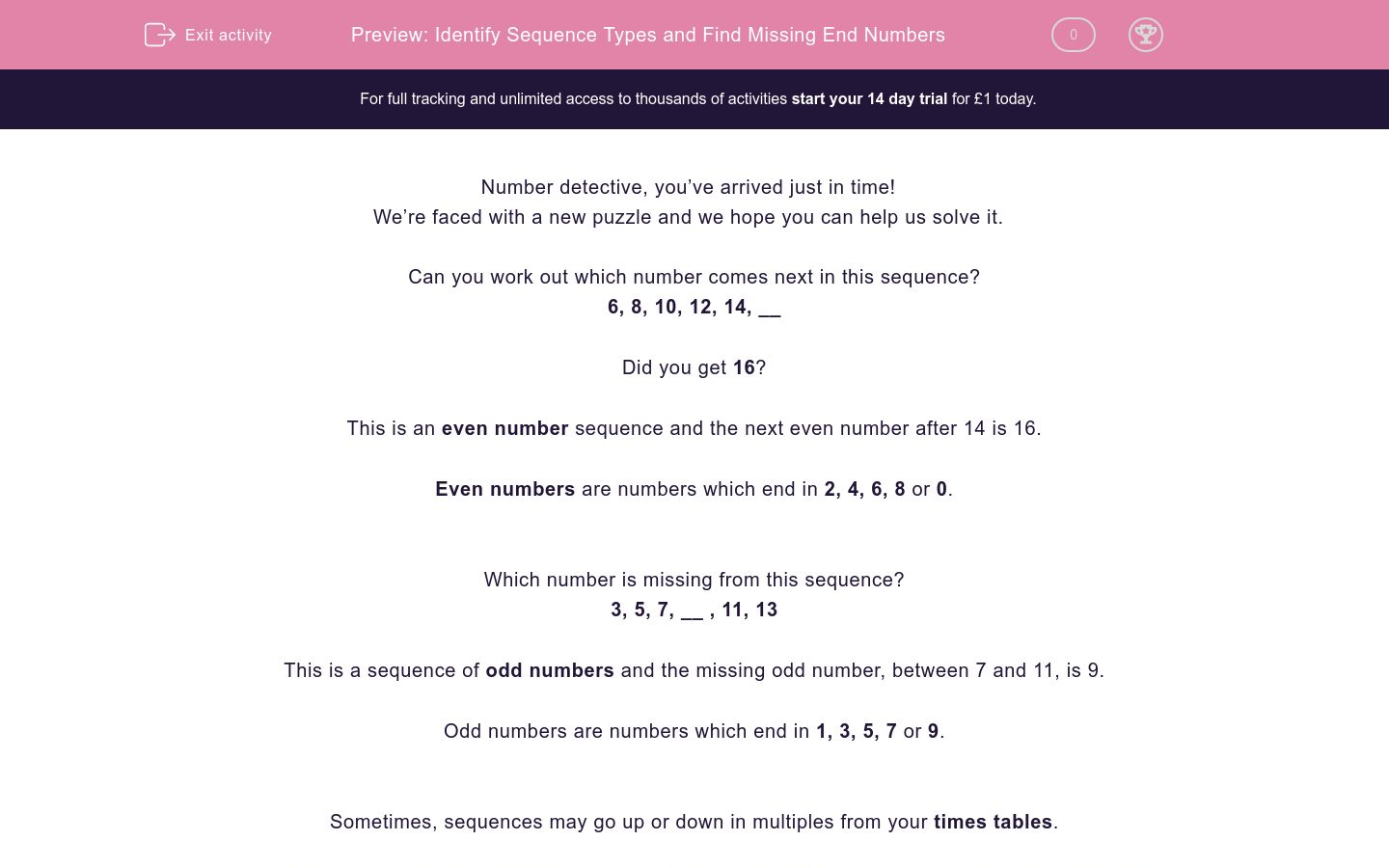# Identify Sequence Types and Find Missing End Numbers

In this worksheet, students will identify missing numbers in sequences of odd and even numbers, as well as times table sequences.Key stage:  KS 2

Curriculum topic:   Verbal Reasoning

Curriculum subtopic:   Number Sequences

Difficulty level:### QUESTION 1 of 10

Number detective, you’ve arrived just in time!

We’re faced with a new puzzle and we hope you can help us solve it.

Can you work out which number comes next in this sequence?

6, 8, 10, 12, 14, __

Did you get 16?

This is an even number sequence and the next even number after 14 is 16.

Even numbers are numbers which end in 2, 4, 6, 8 or 0.

Which number is missing from this sequence?

3, 5, 7, __ , 11, 13

This is a sequence of odd numbers and the missing odd number, between 7 and 11, is 9.

Odd numbers are numbers which end in 1, 3, 5, 7 or 9.

Sometimes, sequences may go up or down in multiples from your times tables.

Which times table does this sequence form part of and can you figure out which number comes next?

3, 6, 9, 12, __

This is the three times table and the missing number is 15.

It’s now your turn to find the missing numbers. Good luck number detective! We are counting on you...

What type of number sequence is this?22, 24, 26, 28, 30

Odd number sequence

Even number sequence

What type of number sequence do we have below?

31, 33, 35, 37, 39

Odd number sequence

Even number sequence

Look at the number sequence below.

Which number would come next?

18, 20, 22, 24, ?Look at the number sequence and fill in the missing number in the blank space below.

Complete the number sequence below by filling in the blank space.

Which number comes next in the following sequence?

Fill in the blank below.

Work out the missing number in this sequence.

70, 80, 90, 100, ?

Which number comes next in the following sequence.

Fill in the blank below.

Match the following sequences to their missing numbers.

## Column B

14, 16, 18, 20
22
15, 20, 25, 30
35
80, 90, 100, 110
120

Match the following sequences to their sequence types.

## Column B

66, 68, 70, 72
5 times table
71, 73, 75, 77
2 times table / even numbers
65, 70, 75, 80
Odd numbers
• Question 1

What type of number sequence is this?22, 24, 26, 28, 30

Even number sequence
EDDIE SAYS
Hi number detective! Did you notice that all of the numbers in this sequence are even numbers? Remember that even numbers end in a 0, 2, 4, 6 or an 8. See you on the next question!
• Question 2

What type of number sequence do we have below?

31, 33, 35, 37, 39

Odd number sequence
EDDIE SAYS
Did you spot our odd numbers here number detective? Odd numbers end in 1, 3, 5, 7 or 9.
• Question 3

Look at the number sequence below.

Which number would come next?

18, 20, 22, 24, ?26
EDDIE SAYS
Did you notice that this sequence was going up in even numbers? The next even number after 24 is 26.
• Question 4

Look at the number sequence and fill in the missing number in the blank space below.

EDDIE SAYS
Did you recognise our odd number sequence here detective? The next odd number after 21 is 23.
• Question 5

Complete the number sequence below by filling in the blank space.

EDDIE SAYS
It's another even number sequence. Even number sequences go up in 2s. Which number is 2 more than 98? 100! Wow, we are into 3 digit numbers now number detective!
• Question 6

Which number comes next in the following sequence?

Fill in the blank below.

EDDIE SAYS
Here our sequence was going up in 5s. The number that is 5 more than 40 is 45. High FIVE for your awesome number detective skills!
• Question 7

Work out the missing number in this sequence.

70, 80, 90, 100, ?

110
EDDIE SAYS
Which times table is this sequence going up in? All of these numbers are in our 10 times table and 10 more than 100 is 110.
• Question 8

Which number comes next in the following sequence.

Fill in the blank below.

EDDIE SAYS
Did you notice that this sequence was going up in 5s? Every multiple of 5 ends in a 5 or a 0. The next multiple of 5 after 70 is 75.
• Question 9

Match the following sequences to their missing numbers.

## Column B

14, 16, 18, 20
22
15, 20, 25, 30
35
80, 90, 100, 110
120
EDDIE SAYS
How many matches did you find? Once you have worked out how much the sequence is going up by each time, you just need to add that number on once more to find the next number in the sequence.
• Question 10

Match the following sequences to their sequence types.

## Column B

66, 68, 70, 72
2 times table / even numbers
71, 73, 75, 77
Odd numbers
65, 70, 75, 80
5 times table
EDDIE SAYS
How many matches could you identify? Make a mental note that even numbers end in 0, 2, 4, 6 and 8 and that odd numbers end in 1, 3, 5, 7 or 9. Use your detective skills to work out how numbers in the 5 times table end. They always end in either a 0 or a 5 - hat's off if you got that one correct!
---- OR ----